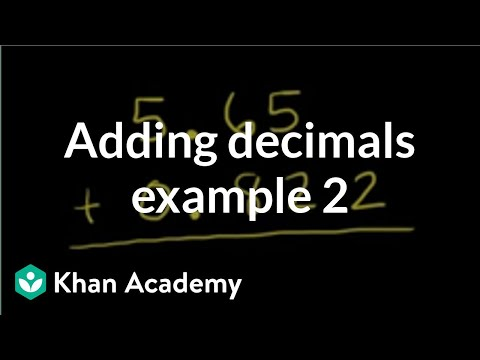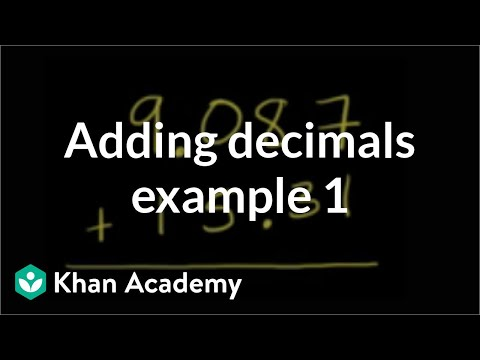COVID-19 presents an unprecedented challenge for teachers, students, and parents. Explore resources from IBM and partners here.
Video

# Dividing decimals with hundredths (Full video)

Description: Decimal divisors in the hundredths place can be a little confusing. In this video we'll show you how to move the decimal and make it a whole number. Pretty nifty! Watch. Created by Sal Khan. Let's see if we can divide 30.24 divided by 0.42. Well the important realization is, is when you're doing a division problem like this, you will get the same answer as long as you multiply or divide both numbers by the same thing. And we know that when we have a fraction like this we're not changing the value of the fraction if we multiple the numerator and the denominator by the same quantity.

### Other videos you might be interested in### Adding decimals: 0.822 + 5.65 (Full video)